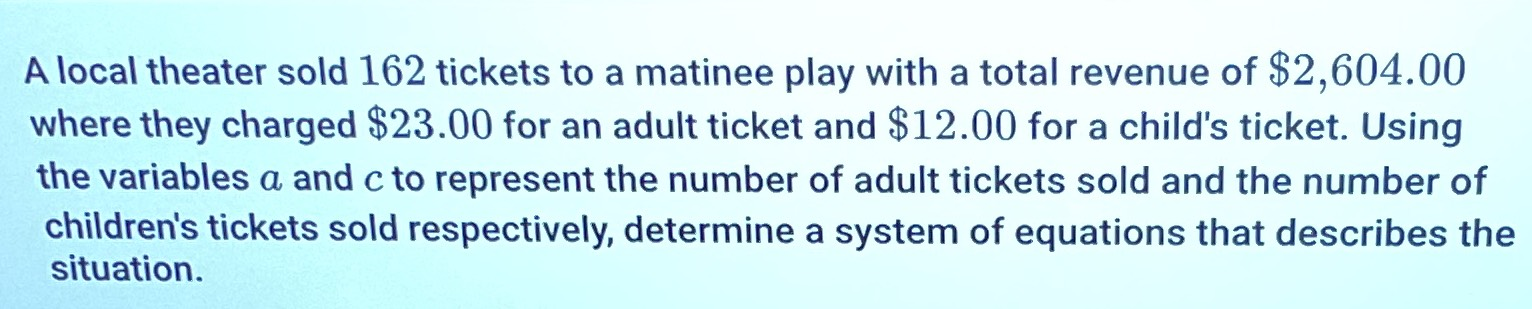### ¿Todavía tienes preguntas de matemáticas?

Pregunte a nuestros tutores expertos
Algebra
PreguntaA local theater sold $$162$$ tickets to a matinee play with a total revenue of $$\ 2,604.00$$

where they charged $$\ 23.00$$ for an adult ticket and $$\ 12.00$$ for a child's ticket. Using the variables $$a$$ and $$c$$ to represent the number of adult tickets sold and the number of children's tickets sold respectively, determine a system of equations that describes the situation.